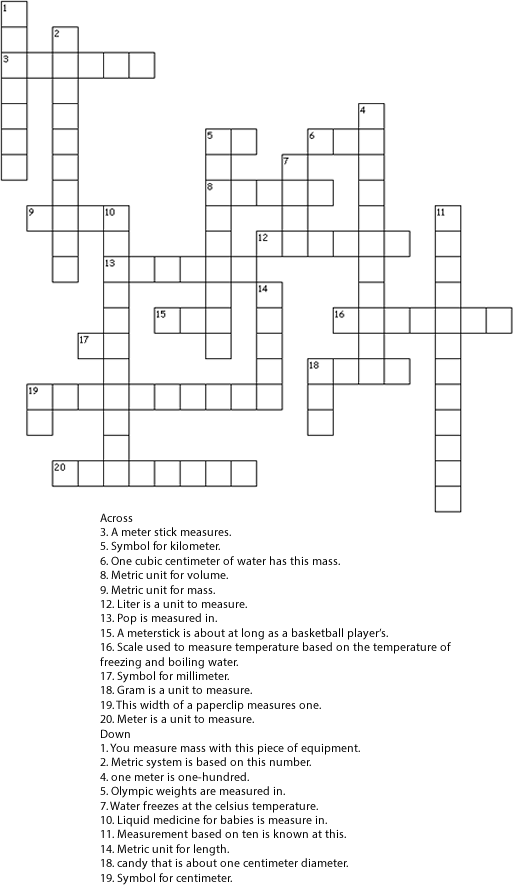# Metric Review

## Short quiz

1. Metric unit for length.
2. Metric unit for mass.
3. Metric unit for volume.
4. Symbol for centimeter.
5. Symbol for meter.
6. Symbol for liter.
7. Symbol for millimeter.
8. Metric system is based on this number.
9. 1000 milliliters is one.
10. Scale used to measure temperature based on the temperature of freezing and boiling water.
11. Water freezes at the celsius temperature.
12. Liter is a unit to measure.
13. Meter is a unit to measure.
14. Gram is a unit to measure.
15. You measure mass with this piece of equipment.
17. This width of a paperclip measures one.
18. Unit to measure distance.
19. Symbol for kilometer.
20. Pop is measured in.
21. 1 meter is 100.
22. 1000 millimeters is one.
23. Liquid medicine for babies is measure in.
24. Olympic weights are measured in.
25. A meter stick measures.
26. A measuring cup measures.
27. One cubic centimeter of water has this mass.
28. Measurement based on ten is known at this.
29. Candy that is about one centimeter diameter.

## Word bank

May use words more than once and their plurals:

m&m
meter, liter, gram, centimeter, milliliters, kilograms
cm, ml, km
one hundred
celsius
zero, one ten, hundred
volume, mass, length
distance
balance
arm
length
volume
metric system

## Crossword puzzleUntitled Document